Functions and CALL Routines

# PVP Function

Returns the present value for a periodic cash flow stream (such as a bond), with repayment of principal at maturity.
 Category: Financial

## Syntax

 PVP(A,c,n,K,k0,y)

### Arguments

A

specifies the par value.

 Range: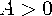c

specifies the nominal per-year coupon rate, expressed as a fraction.

 Range: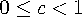n

specifies the number of coupons per year.

 Range: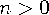and is an integer
K

specifies the number of remaining coupons.

 Range: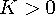and is an integer
k0

specifies the time from the present date to the first coupon date, expressed in terms of the number of years.

 Range: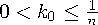y

specifies the nominal per-year yield-to-maturity, expressed as a fraction.

 Range: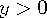The PVP function is based on the relationship
where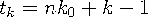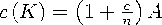```data _null_;
p=pvp(1000,.01,4,14,.33/2,.10);
put p;
run;```

The value returned is 743.168.Previous Page | Next Page | Top of Page